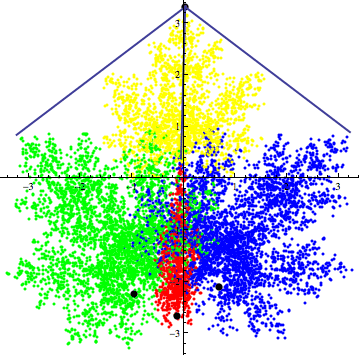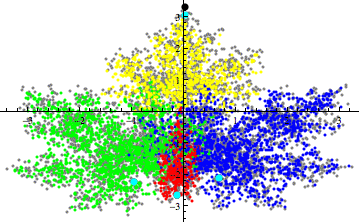Saturday, May 24, 2014

Fractal parameters estimation thru contour analysis - Part I

Introduction
I really love fractals theory. Nothing fascinates me as the amazing structures generated by a chaotic processes that describe (hyperbolic) fractals.
It's fairly easy to draw a fractal image:
• The input
• A small set of very easy function (linear transformations)
• A probability to choose one of the aforementioned functions.
• The process
• Choose randomly a starting point.
• Choose randomly one of the above functions according to the above defined probability.
• Map the starting point using the above selected function.
• iterate as long as you want :)
• Admire the meaning of the life!
• just plot the points
Wherever you are, whatever decision you make, despite the chaos, the overall picture will be always the same. More you will move, more clear the picture will be!

When I approached for the first time fractals,  after the reading of the great Barnley's book (Fractals Everywhere) I opened the laptop and I wrote the routine to draw my first IFS!
...By mistake I also plotted the lines that join the points:

...It's quite a messy, isn't it?
Sometimes it's better to give up to connect the points, just relax and wait until everything will be clear. If we focus on every single detail we risk to lose what really counts!

What is a fractal (Iterated Fractal System)?
There are many formal definitions to describe an IFS, in my opinion the most effective describe a fractal as the fixed point of the Markov process (mentioned above).
The process converges to the fractal :)

The powerful of the fractal
In literature there are plenty articles in which fractals are used to solve complex problems (financial analysis, stock exchange preditictions, description of biological processes,...).
Also in Computer Science fractals fond a good collocation, think about the image compression: the ivy leaf image takes around 25MB of space, all the information to obtain it thru the IFS takes less 1KB!!
The problem
Given the ivy leaf image, what are the parameters of the Markov process to generate it?
A bit more formally what we have to estimate is:
• A set of functions: $\{ \tau_1, \cdots,\tau_N \}$, where $\tau_i(p_0)=A_1 \cdot p_0 +Q_1$, where:
$A= \begin{bmatrix}\alpha & \beta\\ \gamma & \delta \end{bmatrix} Q= \begin{bmatrix} \epsilon\\ \zeta \end{bmatrix}$
• We need also the estimate the probability to choose each $\tau_j$
The combination of the collage theorem and the box counting approach is the most common technique to solve the problem.
When I was student I approached the problem from a different angle. I have to say that the results obtained were partials but I still think that something more can be done :)
Before to start we need one more notion: the contractive maps (have a look at Banach Theorem)
Under certains conditions the iteration  $\tau_j$ led to a fixed point:Example of contractive map applied to an ellipsoid. It converges to a fixed pointed.

First conjecture:

• An IFS is characterised by a fixed point that lies on its convex hull.
• From a fixed point that lies on the border of the IFS, the iterations of the contractive maps that generate the fractal return the convex hull of the fractal.The ivy leaf IFS is generated by 4 contractive maps. Each color describes the map used to generate the point.
The above animated gif shows the meaning of the conjecture.
An experimental evidence about the fact that at least one fixed point lies on the convex hull of the fractal can be obtained changing the params of the maps:The light blue points depict the fixed point of the maps used to plot the fractals.
Despite the changes in the maps, the fixed point on top of the leaf still lays on the convex hull of the fractal.
In the next post I'll show you a nice recursive algorithm I found to obtain different levels of convex hull for an IFS.
Stay tuned
Cristian.

Friday, May 2, 2014

Waiting Time Polynomials: tech explanation - last part

This is the last step to complete the explanation of the waiting time polynomials formula.

Unfortunately it's a bit technical, but I'm sure that it can be understood without deep math knowledge.
At the very end if you can't explain it simply you don't understand it well enough! (A. Einstein)

The trick
Last time we left with the tally of overall waiting time $w(x_i) = \phi(x_i)-|x_i|$ where $\phi(x_i)$ returns just the position of the last $|x_i|$ in the vector $V$.
Let's have a look at the following example that will be used during the remaining steps.
There are two questions that might be answered:

• given $|x_1|= i, |x_2|= j, |x_3|= k$ what are the tuples $\{w(x_1),w(x_2),w(x_3)\}$?
• given a $\{w(x_1)=I,w(x_2)=J,w(x_3)\}=K$, how many vectors $V$ can be built?
To answer to the first question, I noticed that the three coloured cells are the only ones that really count.
The idea is the following:

1. consider the three cells as placeholders
2. analyse the admitted values for each of them
3. replace the placeholders with all the possible permutations of the alphabet $\{x_1,x_2,x_3\}$.

Let's start with the case depicted in the above image, where we assumed that $\phi(x_1) < \phi(x_3) < \phi(x_2)$, then we have the following facts:
• $\phi(x_1)$ can take values between: $0 \leq \phi(x_1) \leq |x_1|+|x_2|+ |x_3|-2$
• $\phi(x_2)$ can take just one value: $|V|=|x_1|+|x_2|+ |x_3|$
• The upper bound of $\phi(x_3)$ is $|V|-1$ because it can slide till the second last element of $V$, that is $\phi(x_2)-1$
• what about the lower bound of $\phi (x_3)$? We have two cases depicted in the below image:

To sum up, so far we explained the following part of the formula (I hope you don't mind that I changed a bit the indexes notation):

We have now to consider that for each configuration of $\{w(x_1)=I,w(x_2)=J,w(x_3)\}=K$ we can have more than one vector $V$.
Do you like combinatorics? The remaining part it's just matter of tally, and the only formula we need is the formula for permutation with repetitions. I let you rub up the concept on your favourite website for trivial combinatorics.

The formula can be split in two chunks, because we have to blocks of cells to be filled

1. In how many ways can we fill the cells between the positions $[1,\phi(x_1)]$?
2. In how many ways can we fill the cells between the positions $[phi(x_1),phi(x_2)]$?

Let's answer the first question we have to find the values for the denominator of the following:
$\frac{(\phi(x_1)-1)!}{\#(x_1)!\#(x_2)!\#(x_3)!}$
• we have $|x_1|-1$ cells that can be filled.
• it contains all the instances of $x_1$ (except for the last the occupied $\phi(x_1)$)
• the number of $x_3$ instances depends by $\phi(x_1)$ and $\phi(x_3)$:

• the computation of the number of instances of $x_2$ in the first slot is straightforward, and it can easily derived by difference:
• $\frac{(\phi(x_1)-1)!}{(|x_1|-1)!(|x_3|-Min(|x_3|,\phi(x_3)))!\#(x_3)!}$;
• $(|x_1|-1)+(|x_3|-Min(|x_3|,\phi(x_3)))+\#(x_2)=\phi(x_1)-1$
• so $\#(x_2)= \phi(x_1)-|x_1|-(|x_3|-Min(|x_3|,\phi(x_3)))$
This explains the following boxed part of the formula:

The final step is to count in how many ways we can fill the slot 2 depicted by the interval $[phi(x_1),phi(x_2)]$ and to make the formula more readable let's rename $(|x_3|-Min(|x_3|,\phi(x_3))= \epsilon$.
As we did for the first slot we have to identify the values of the denominator of the below formula:
$\frac{(\phi(x_3)-\phi(x_1)-1)!}{\#(x_2)!\#(x_3)!}$

• Out of $|x_3|$ instances, $\epsilon$ have been placed in the slot 1, so the slot 2 contains exactly $|x_3|-1- \epsilon$.
• again by difference we can get the instances of $x_2$:
• the occurrences of $x_2$ before $\phi(x_3)$ are exactly $\phi(x_3)- (|x_1|+|x_3|)$
• the occurrences of $x_2$ in the slot 1 (listed above) are: $\#(x_2)= \phi(x_1)-|x_1|-\epsilon$
• that is : $\#(x_2)=\phi(x_3)- (|x_1|+|x_3|)- \phi(x_1)+|x_1|+ \epsilon$
• finally we have: $\#(x_2)=\phi(x_3)-\phi(x_1)-|x_3|+ \epsilon$

That's complete the proof of the formula.
It's quite easy now extend the formula to more variables. The only expedient to make it easier is to remove from the formula the operator $Min$ splitting the formulas in two branches.
I'll show it in paper.
... The introduction of placeholders requires to apply the formula for each permutation of the variables involved. It means that having $k$ variables we need to calculate the formula $k!$